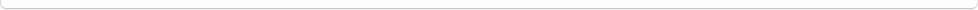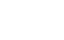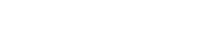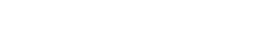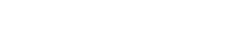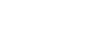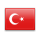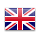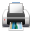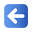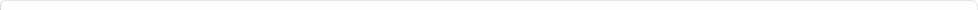Description of Individual Course Units
 Course Unit Code Course Unit Title Type of Course Unit Year of Study Semester Number of ECTS Credits 180102003100 DIFFERENTIAL EQUATIAONS Compulsory 1 1 5
Level of Course Unit
First Cycle
Objectives of the Course
To introduce the purpose of differential equations and their applications in engineering. To interpret the results by making solutions of different types of differential equations.
Name of Lecturer(s)
Dr.Öğr.Üyesi Gökçe Dilek KÜÇÜK
Learning Outcomes
 1 Define the concept of differential equation. 2 Define the concept of differential equation. 3 Solve some types of differential equations with first order and first order. 4 Solve some types of differential equations with first order and first order. 5 Solve some types of differential equations with first order and higer degree 6 Solve some types of differential equations with first order and higer degree 7 Be able to establish a differential equation of some physical problems. 8 Be able to establish a differential equation of some physical problems. 9 Gain the ability to interpret some differential equations encountered in engineering 10 Gain the ability to interpret some differential equations encountered in engineering
Mode of Delivery
Daytime Class
Prerequisites and co-requisities
Recommended Optional Programme Components
Course Contents
Weekly Detailed Course Contents
 Week Theoretical Practice Laboratory 1 Definition and Classification of Differential Equations 2 Derivation of Differential Equations. 3 Separable Differential Equations 4 Homogeneous Differential Equations 5 Exact Differential Equation 6 Becomes Exact Differential Equations That can be Converted 7 Linear Differential Equations, Bernoulli Differential Equations 8 Mid-Term Exam 9 Homogeneous Differential Equations with Constant Coefficients 10 Non-homogeneous Differential Equations with Constant Coefficients 11 Laplace transform. Laplace transform of derivative. Inverse Laplace Transform. Engineering Applications. 12 First Order Linear Differential Equation Systems 13 Homogeneous Linear Equation Systems with Constant Coefficients 14 Non-homogeneous Differential Equation Systems with Constant Coefficients 15 Introduction to partial differential equations 16 Final Exam
Recommended or Required Reading
Planned Learning Activities and Teaching Methods
Assessment Methods and Criteria
 Term (or Year) Learning Activities Quantity Weight Midterm Examination 1 100 SUM 100 End Of Term (or Year) Learning Activities Quantity Weight Final Examination 1 100 SUM 100 Term (or Year) Learning Activities 40 End Of Term (or Year) Learning Activities 60 SUM 100
Language of Instruction
Turkish
Work Placement(s)